# Mathematics - Mathematical Analysis Surface and Contour Integrals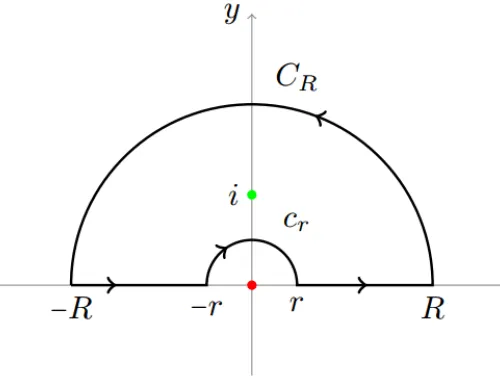Source

## Introduction

Hello it's a me again drifter1!

Today we continue with Mathematical Analysis by getting into Surface and Contour Integrals. By doing that we will also talk about polar coordinates, the Jacobi determinant, shapes, vector functions, theorems and more...And so this post will contain a lot of interesting stuff about them!

Also I suggest you reading my post about Double integrals so that you have some knowledge about them, cause the Integrals that we will discuss today are special cases of them!

So, without further do, let's get started!

## Small recap

In the previous post we talked about Double Integrals and how they are useful.

For a plane/area/surface R and a function f(x, y) we define:
quicklatex

which are the equations for:

• the Volume of the projection of f(x, y) into the area R
• and by setting f(x, y) = 1 we also then get the actual area R

We can also have Multiple-Integrals that can also be in more then 2 or 3 dimensions (and so variables), but then there is a little problem. We can't actually perceive it in space and so understand it correctly, but this doesn't mean that they are not useful...

## Polar coordinates and Jacobi determinant

Sometimes such an Surface Integral can be difficult to solve.

Even when having 2 variables and so being able to change the "order" we integrate in, we again may have a problem calculating it, cause the functions are not so simple.

A way of making some integrals easier and a way of representing some shapes with a smaller and more efficient function is by changing the parameters/variables we refer to.

For example we can use Polar coordinates that take us from (x, y) to (r, θ).

That way we leave the classic Cartesian space to go to another space where all the points are represented using the radius r (the distance from the center O) and the angle θ (the angle corresponding to the x'x axis).Source

Using the trigonometric functions sinθ and cosθ, x and y are:

x = rcosθ

y = rsinθ

where 0 <= θ <= 2π

To change the variables we have to use the following formula: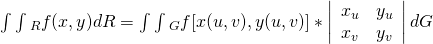quicklatex

where the last part of the second integral is the Jacobian determinant.

In the Jacobian determinant each row contains the partial derivatives of x, y with the corresponding "new" variable.

u, v are of course r, θ in our case, but I wanted to stay with the actual formula.

This means that:

xr = ∂(rcosθ)/∂r = cosθ

yr = ∂(rsinθ)/∂r = sinθ

xθ = ∂(rcosθ)/∂θ = -rsinθ

yθ = ∂(rsinθ)/∂θ = rcosθ

The value of a 2x2 determinant is equal to the cross-product and so:

cosθ*rcosθ - sinθ*(-rsinθ) = rcos^2θ + rsin^2θ = r(sin^2θ + cos^2θ) = r

And so when having polar coordinates the formula becomes:where t == θ (quicklatex doesn't let me use greek characters)

Very easy to remember that we just multiply our function with r and change the upper and lower bounds of the definite integrals :)

## Interesting Shapes in the Polar coordinate System

Using this Polar coordinate system we can represent the following shapes...

Circles: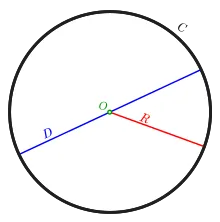Source

Which are the most essential and simplest shape in this space.

The equation of any circle is:

x^2 + y^2 = r^2

Cardiods (hearts):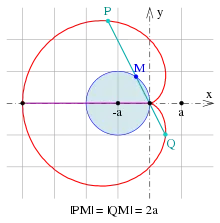Source

Which follow the equation: r(θ) = a(1 +- cosθ) or r(θ) = a(1 +- sinθ)

And also the famous Bernoulli equation:

r^2 = a^2*cos(2θ)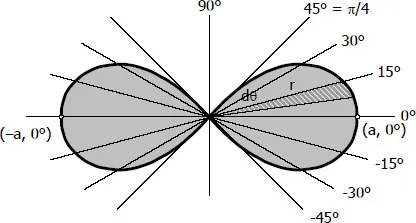Source

And you can find even more...

## Contour Integrals of Vector functions

Suppose we now have the vector function:

F(x, y, z) = M(x, y, z)i + N(x, y, z)j + P(x, y, z)k = (M, N, P)

And we also have the curve:

c: r(t) = x(t)i + y(t)j + z(t)k, t in a range [a, b]

The following integral: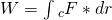quicklatex

is called the contour integral (or integral on top of the curve) of F along the path of curve c.

F*dr is of course a dot product between two vectors: F(M, N, P) and dr = (dx, dy, dz).

Spoiler, spoiler!
We will use this for calculating the Work/Energy of a Force across a path in Physics soon! :)

Continuing on with the "expansion" of this integral we get: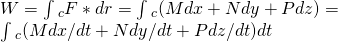quicklatex

Which you might recognize as the tangent vector dr/dt of the curve c = r(t).

So, when F is refering to points on top of curve c then M, N, P become functions of t, cause x = x(t), y = y(t) and z = z(t). That way: M(x, y, z) = M(x(t), y(t), z(t)), and the same for N and P...

This means that it's very easy to calculate such an integral :P

A very interesting Property of such Integrals is the following...

When c is a curve that is made up of many other curves c1, c2, ..., cn so that each one's end is the start of the other then:quicklatex

## Green and Stoke Theorems

Some of those curves might be closed "circles".

When that happens then we can use Green's theorem that tells us:

If F(x, y) = M(x, y)i + N(x, y)j is a vector function

and

c: r(t) = x(t)i + y(t)j a closed curve that encloses a area R

Then:Source

Which means that we can "leave" the contour integral and go to an surface integral that might be easier to solve.

Stoke's theorem is somewhat similar, but gets us back to the Del of dr/dt which is the vector that we get by using only the partial derivatives for each axis.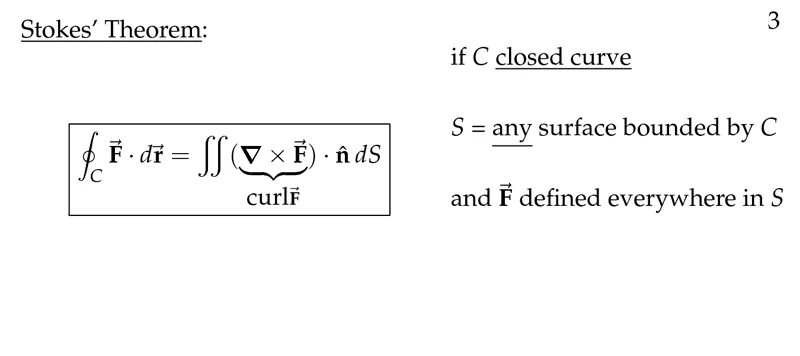Source

Both of those theorem's are very useful and you should be using them anytime you find difficulties when calculating one or the other type of integral :)

And this is actually it for today and I hope that you enjoyed it!

Next time we will get into a lot of Examples for everything!

Bye!

H2
H3
H4
3 columns
2 columns
1 column
Join the conversation now